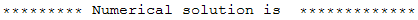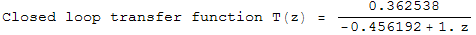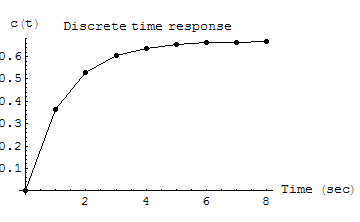A Script for Symbolic and Numerical Solution of System Time Response for a Simple Closed Loop Discrete Time System

by Nasser Abbasi
May 11, 2005.

This is a simple Mathematica script which takes as input the reference signal R(s), the plant transfer function G(s), and the sample time T, and generate the sampled output c[kT]. The intermediate computation of G(z) and the closed loop transfer function T(z) and C(z) and c[KT] are all shown both in symbolic format and in numerical format.

This script solves only for this system configuration, for arbitrary G(s), T, and R(s).To illustrate, the script is run to solve examples 6.1 and example 6.4 in the text book Digital Control System Analysis and Design, 3rd edition by Phillips and Nagle. The result generated matches the text book result with the additional advantage of being able to see the analytical steps taken during the solution steps.
Next I need to add the ability to generate the solution c(t) to the sampled input.

Data Input section

Here type in the input for the system (this could also be done via an Input statement if needed) for now, simply edit these lines to change the input as needed.Example 6.1 outputExample 6.4 outputCode sectionCreated by Mathematica  (March 4, 2006)